# Transformer Inrush Current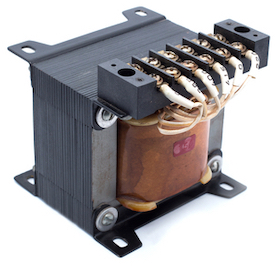Figure 1: Transformer

Transformer inrush current is the instantaneous current drawn by the primary winding of the transformer when the transformer is initially energized with the secondary side left open-circuited. The inrush current also referred to as magnetizing inrush current, is transient in nature and exists only for a few milliseconds. The inrush current is two to ten times higher than the standard rated current of the transformer. This article discusses the basic definition of transformer inrush current, its causes, effects, and remedies.

## What is transformer inrush current?

The transformer inrush current is the instantaneous current drawn by the primary winding of the transformer when the transformer is energized with the secondary side left open-circuited. The secondary side of a transformer is energized only by the magnetic flux from the primary side. Therefore there is no current flow in the secondary windings (hence open-circuited for a very short while) at the moment the transformer’s primary winding is energized.

The inrush current does not create any permanent fault in the transformer. Still, it causes an unwanted switching in the circuit breaker (an electrical switch designed to protect an electrical circuit from damage caused by a short circuit or overcurrent).

During the inrush current, the maximum value attained by the magnetic flux is over twice the normal flux. The magnitude of inrush current (Figure 2 labeled A) is 2-10 times that of the transformer full load current (Figure 2 labeled B). Toroidal transformers that use less copper for the same power handling can have up to 60 times the inrush to running current.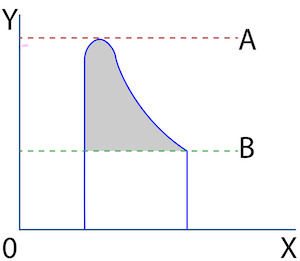Figure 2: Transformer peak current (A) and steady-state current (B)

## How to calculate transformer inrush current

Use the following equation to calculate the inrush current in a transformer:

Ip = 1.414 Vm / R

• Ip: Transformer inrush current
• Vm: Peak magnitude of applied voltage
• R: DC winding resistance

### Example

To calculate the transformer inrush current of a 100kVA, 440V transformer that has a DC winding resistance of 0.5 ohms:

• Vm = 440V
• R= 0.5 ohms
• Therefore, Ip = (1.414 × 440) / 0.5 = 1244.3 Amperes

Full-load current of the transformer= (KVA × 1000) / Vm =227A.

Therefore, the transformer inrush current is approximately six times that of the full load current.

## How to measure transformer inrush current

A clamp meter can be used to measure the inrush current in a transformer. A clamp meter measures current through a device without making physical contact. The non-contact feature ensures a safe working option for measuring very high currents, which can otherwise be hazardous to the user. Read our article on how to use a clamp meter for more information.

## Transformer inrush current cause

When an alternating voltage is applied to a transformer whose secondary side is open-circuited, the device acts as a simple inductance. The current passes through the primary winding when a transformer is energized through an alternating voltage. According to Faraday’s law of induction, the current creates a magnetic flux around the primary winding. The magnetomotive force (MMF) drives the flux through the core, and its value is proportional to the current through the winding. The MMF is given by:

MMF = N × I

• MMF: Magnetomotive force
• N: Number of turns
• I: Winding current

A part of the magnetic flux passes through the core and links with the secondary winding through mutual induction, initiating a current flow on the secondary side. Read our article on electrical transformers for more information on how transformers work.

The rate of change of magnetic flux in a transformer core is proportional to the instantaneous voltage drop across the primary winding.

V = dɸ / dt

ɸ = Integral ( V )

• V: Applied AC voltage
• ɸ: Magnetic flux produced

In a continuously-operating transformer, the MMF is proportional to the winding current. Since the flux is proportional to the MMF, the current and flux remain in phase with each other. Since voltage leads current by 90 degrees in an inductor, it can be deduced that voltage leads magnetic flux by 90 degrees (or quarter cycle), as seen in Figure 3.

As per the waves shown in Figure 3, at the instant when the voltage (Figure 3 labeled V) is zero, the corresponding steady-state flux (Figure 3 labeled F) value should be the negative maximum (i.e., minimum value). But there is no flux linked to the transformer core before switching on the power supply. It is practically impossible to have flux the instant the supply is switched on. The steady-state flux value can not be reached instantly and takes a non-zero amount of time.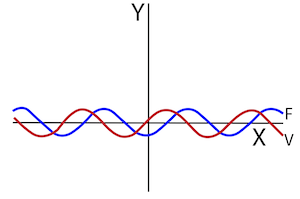Figure 3: The flux (F) starts from a negative peak when the applied voltage (V) starts at zero

Hence, If the transformer is switched on at the instant when the voltage is zero, the flux wave starts from the same origin as the voltage waveform, as seen in Figure 4. The flux value at the end of the voltage waveform’s first half cycle can be calculated using:• ɸmax: Maximum magnetic flux created
• E sin⍵t: Alternating voltage applied at primary side
• E: Magnitude of the applied voltage
• ⍵t: Phase of the applied voltage

The transformer core is saturated above the maximum steady-state flux value (Figure 4 labeled B). The core becomes saturated after the maximum flux value, and the current required to produce the rest of the flux is very high. Therefore the primary winding of the transformer draws a very high peak current from the source known as the transformer inrush current (Figure 4 labeled A) or magnetizing inrush current of the transformer. This transient current exists for a few milliseconds, after which it settles down.

The transformer inrush current exists for a very short time; hence this current does not create any permanent faults in transformers. However, it interferes with the operation of circuits connected to the transformer causing unnecessary switchings and voltage transitions.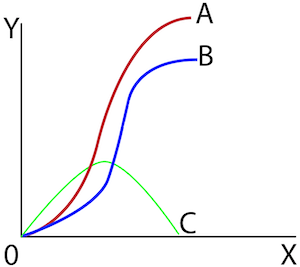Figure 4: Transformer inrush current (A), maximum flux generated (B), and applied alternating voltage (C)

## Effects of transformer inrush current

1. High magnetizing inrush current in transformer necessitates over-sizing of breakers or fuses.
2. Injection of distortion and noise back into the main power supply.
3. Arcing (electric current jumping from one connection to the other) and failure of circuit components like switches.
4. Interrupted operation in fuse.
5. Current disturbance and harmonics in the system and lower power quality characteristics.
6. Irregular voltage distribution along the transformer windings.
7. Mechanical and electrical vibrations along the transformer windings.

## How to reduce transformer inrush current

The following methods can reduce the inrush current in a transformer:

### Adjusting the input voltage phase

Transformer inrush current occurs if the alternating voltage reaching the transformer primary winding starts at zero when the transformer is energized. If the phase of the incoming voltage begins at 90 degrees (maximum amplitude), the corresponding value of current will be zero as current lags voltage by 90 degrees in an inductor. Hence, adjusting the phase angle of the input voltage to reach the transformer's primary winding at its positive peak value is a viable solution, as seen in Figure 5.

However, the inrush current lasts for only a few milliseconds, and the transformers are physically designed to handle the mechanical stresses from to the inrush current. Adjusting the applied voltage's phase angle to reduce inrush current is typically not necessary or economical. A simple solution is to use a phase meter to monitor the phase of the incoming voltage and apply the voltage to the transformer only when it is at maximum value. Switching the supply at the proper instant can reduce the magnitude of the transient inrush current.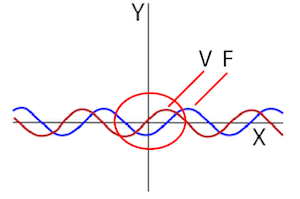Figure 5: The flux (F) starts from zero when the applied voltage (V) begins at a positive peak

### Use an inrush current protection circuit

Thermistors can be used to limit the high starting current in a transformer. A thermistor has a very high resistance at ambient temperatures and low resistance at high temperatures. The thermistor is connected in series with the power supply input line. So, when the device is turned on, the high resistance limits the inrush current to flow into the system. As the current flows continuously, the thermistor temperature rises, which reduces the resistance significantly. Hence, the thermistor stabilizes the inrush current allowing the steady current to flow into the circuit.

Therefore, adjusting the phase of the incoming voltage or adding a protection circuit to the transformer can decrease the inrush current spike, as seen in figure 6.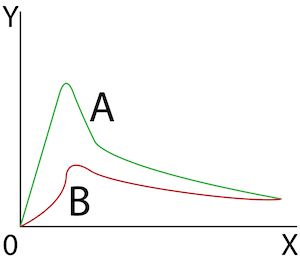Figure 6: Inrush current (A) and reduced inrush current (B)

## FAQs

### What is transformer inrush current?

Transformer inrush current describes a spike in current that occurs when the transformer is initially turned on.

### What causes transformer inrush current?

When a transformer is energized, the transformer may draw a large inrush current from the system due to the magnetic flux in the core being out of phase with the applied voltage.

### How long does the transformer inrush current last?

The transformer inrush current is transient in nature and can last up to a few milliseconds.